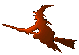Hex  1.0 Hydrogen-electron collision solver
CsrMatrix Class Reference

Complex CSR matrix. More...

#include <matrix.h>

## Data Structures

class  LUft
LU factorization object. More...

class  PngGenerator

## Public Member Functions

CsrMatrix ()

CsrMatrix (size_t m, size_t n)

CsrMatrix (CsrMatrix const &A)

CsrMatrix (size_t m, size_t n, lArrayView const &p, lArrayView const &i, cArrayView const &x)

~CsrMatrix ()

void drop ()
Clear data. More...

cArray dot (cArrayView const &b) const

size_t size () const

size_t rows () const

size_t cols () const

lArray const & p () const

lArray const & i () const

cArray const & x () const

double norm () const

void write (const char *filename) const

void plot (const char *filename, double threshold=0.) const

LUft factorize (double droptol=0) const
Compute (incomplete) LU factorization. More...

cArray solve (const cArrayView b, size_t eqs=1) const
Solve the Ax = b problem, where "b" can be a matrix. More...

void link (std::string name)
Link to a disk file. More...

Complex operator() (unsigned i, unsigned j) const

CsrMatrixoperator*= (Complex r)

CsrMatrixoperator&= (CsrMatrix const &B)

CsrMatrixoperator^= (CsrMatrix const &B)

CsrMatrix sparse_like (CsrMatrix const &B) const

cArray diag () const

CooMatrix tocoo () const

RowMatrix< Complextorow () const

cArray upperSolve (cArrayView const &b) const

cArray lowerSolve (cArrayView const &b) const

template<class Functor >
CsrMatrix nzTransform (Functor f) const

bool hdfsave () const

bool hdfsave (std::string name) const

bool hdfload ()

bool hdfload (std::string name)

## Detailed Description

Complex compressed-storage-row-major-ordered sparse matrix. The data are stored in four arrays,

* p, i, x, z
*

Here, Ap is an array of N+1 elements, where N is the number of rows. It contains indices of beginnings of single rows in all remaining arrays. The last element contains one-past-end pointer, i.e. it should be aqual to the element count of the matrix (zero and nonzero). The array Ai holds column indices of elements, which are stored in Ax and Az, real ang imaginary part being separated.

## Constructor & Destructor Documentation

 CsrMatrix::CsrMatrix ( )
inline
 CsrMatrix::CsrMatrix ( size_t m, size_t n )
inline
 CsrMatrix::CsrMatrix ( CsrMatrix const & A )
inline
 CsrMatrix::CsrMatrix ( size_t m, size_t n, lArrayView const & p, lArrayView const & i, cArrayView const & x )
inline
 CsrMatrix::~CsrMatrix ( )
inline

## Member Function Documentation

 size_t CsrMatrix::cols ( ) const
inline
 cArray CsrMatrix::diag ( ) const

Return dense array with diagonal elements of the matrix.

 cArray CsrMatrix::dot ( cArrayView const & b ) const

Ordinary matrix-vector product, $$A\cdot b$$.

Parameters
 b Vector to multiply with.
 void CsrMatrix::drop ( )
inline
 CsrMatrix::LUft CsrMatrix::factorize ( double droptol = 0 ) const

This function computes the LU factorization of the matrix. It uses the free UMFPACK library.

Parameters
 droptol Drop tolerance. If an element of the factorization matrices should be in absolute value smaller than "droptol" the library will omit it completely effectively making the result more sparse.
Returns
Special structure of the LUft type, holding opaque information about the factorization.
 bool CsrMatrix::hdfload ( )
inline

Load matrix from HDF file.

 bool CsrMatrix::hdfload ( std::string name )
 bool CsrMatrix::hdfsave ( ) const
inline

Save matrix to HDF file.

 bool CsrMatrix::hdfsave ( std::string name ) const
 lArray const& CsrMatrix::i ( ) const
inline
 void CsrMatrix::link ( std::string name )

Set a default I/O file that will be used if any of the functions hdfload or hdfsave will be used without an explicit filename.

 cArray CsrMatrix::lowerSolve ( cArrayView const & b ) const

Solves lower triangular system of equations using backsubstitution. The matrix needs not be triangular, but elements above the diagonal won't be used or changed.

Parameters
 b Right-hand side.
 double CsrMatrix::norm ( ) const
template<class Functor >
 CsrMatrix CsrMatrix::nzTransform ( Functor f ) const
inline

Applies a user transformation on nonzero matrix elements.

Parameters
 f A functor compatible with following declaration: * Complex (*f) (size_t i, size_t j, Complex z); *
 CsrMatrix & CsrMatrix::operator&= ( CsrMatrix const & B )

Addition of another CSR matrix. The matrices MUST HAVE THE SAME SPARSE STRUCTURE, as no indices are checked.

 Complex CsrMatrix::operator() ( unsigned i, unsigned j ) const

Element-wise access (const). If a non-existing element is referenced, zero is returned.

 CsrMatrix & CsrMatrix::operator*= ( Complex r )

Multiplication by a number.

 CsrMatrix & CsrMatrix::operator^= ( CsrMatrix const & B )

Subtraction of another CSR matrix. The matrices MUST HAVE THE SAME SPARSE STRUCTURE, as no indices are checked.

 lArray const& CsrMatrix::p ( ) const
inline
 void CsrMatrix::plot ( const char * filename, double threshold = 0. ) const

Save matrix structure as a black-and-white image.

Parameters
 filename File name. threshold Largest absolute value represented by white colour.
 size_t CsrMatrix::rows ( ) const
inline
 size_t CsrMatrix::size ( ) const
inline
 cArray CsrMatrix::solve ( const cArrayView b, size_t eqs = 1 ) const

Uses UMFPACK through the LUft::solve function.

Parameters
 b Complex vector containing column-major ordered data; it may be a flattened matrix. eqs Number of columns.
Returns
Array of roots in the same shape as "b".
 CsrMatrix CsrMatrix::sparse_like ( CsrMatrix const & B ) const

Sets fill-in elements so that the storage structure of this matrix will be identical to that of the other CSR matrix. We can the use “fast” arithmetic operators & and ^.

Returns
self
 CooMatrix CsrMatrix::tocoo ( ) const

Convert to COO format.

 RowMatrix< Complex > CsrMatrix::torow ( ) const

Convert to dense matrix.

 cArray CsrMatrix::upperSolve ( cArrayView const & b ) const

Solves upper triangular system of equations using backsubstitution. The matrix needs not be triangular, but elements under the diagonal won't be used or changed.

Parameters
 b Right-hand side.
 void CsrMatrix::write ( const char * filename ) const

Write the matrix data to a file.

Parameters
 filename Filename.

Format of the fields i,j,x,z is:

* %d\t%d\t%g\t%g
*
 cArray const& CsrMatrix::x ( ) const
inline

The documentation for this class was generated from the following files: